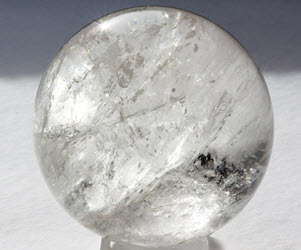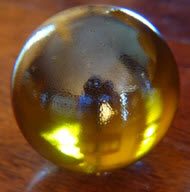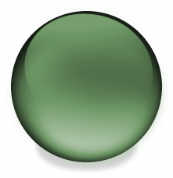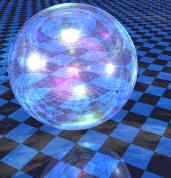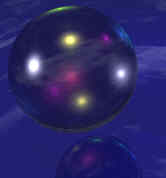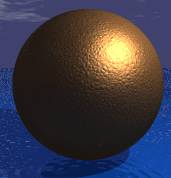# 球体• 完全对称
• 表面上所有的点
与球心的距离 "r" 是相等的
• 没有边或
顶点（角）)
• 有一个表面
（不是 "平面"）
• 不是多边形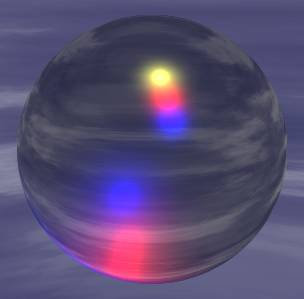## 最大的体积，最小的表面积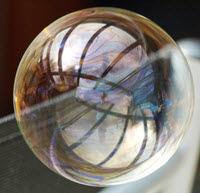## 地球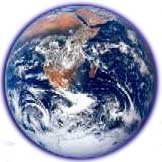## 体积和表面积### 例子：r = 5

 表面积 = 4 × π × r2 = 4 × π × 52 = 4 × π × 25 = 100 π ≈ 314

### 例子：r = 5

 体积 = (4/3) × π × r3 = (4/3) × π × 53 = (4/3) × π × 125 = (500/3) × π ≈ 524

## 酷球体